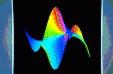# BRIGITTE VALLÉE'S RESEARCH, PREPRINTS, AND REPRINTSThis webpage describes my research in a detailed manner until 2007. For a description of my recent results since then, and a list of publications, see here.

Ages ago, I started my career in the Department of Mathematics at the University of Caen. My interests then gradually shifted from "pure" number theory to computational number theory and related problems in cryptography, most notably integer factorization and lattice reduction. In this area, I obtained average-case bounds for the celebrated LLL algorithms and once held the world record for the best proven bound on the complexity of integer factorization. Currently the core of my work deals with analysis of algorithms. I am especially interested in applications of dynamical systems theory to some of the major algorithms and data structures studied by theoretical computer scientists. I feel for instance responsible for the introduction of transfer operators in this area. My most recent contributions deal with the average-case analysis of lattice reduction in two or more dimensions, the exact analysis of a rich class of continued fraction algorithms, the application of transfer operators to analytic information theory, and the related analysis of digital trees ("tries") under nonuniform input models.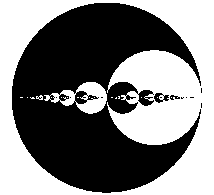My personal research logo: the discs associated with continued fraction expansions of real numbers.2007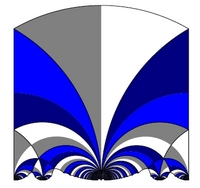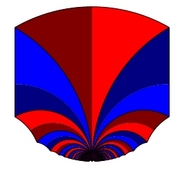Festoons appearing in the analysis of lattice reduction.

• Lattice Reduction in Two Dimensions: Analyses under Realistic Probabilistic Models. By Brigitte Vallée and Antonio Vera. Version of March 2007; submitted to AofA07 (International Conference on Analysis of Algorithms).

The Gaussian algorithm for lattice reduction in dimension 2 is precisely analysed under a class of realistic probabilistic models, which are of interest when applying the Gauss algorithm "inside" the LLL algorithm. The proofs deal with the underlying dynamical systems and transfer operators. All the main parameters are studied: execution parameters which describe the behaviour of the algorithm itself as well as output parameters, which describe the geometry of reduced bases.2006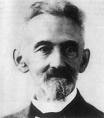• Hausdorff Dimension of Real Numbers with Bounded Digit Averages. By Eda Cesaratto and Brigitte Vallée. Version of August 2006, In Acta Arithmetica.

This paper considers numeration schemes, defined in terms of dynamical systems and studies the set of reals which obey some constraints on their digits. Classically, the studied constraints involve each digit in an independent way. Here, more conditions are studied, which only provide (additive) constraints on each digit prefix. The main example of interest deals with reals whose all the digit prefix averages in their continued fraction expansion are bounded by M. We first provide a characterization of the Hausdorff dimension $s_M$, in terms of the dominant eigenvalue of the weighted transfer operator relative to the dynamical system. We then come back to our main example; with the previous characterization at hand and use of the Mellin Transform, we exhibit the behaviour of |s_M-1| when the bound \$M\$ becomes large.

• Pattern matching statistics on correlated sources. by Jérémie Bourdon and Brigitte Vallée. In LATIN 2006, pages 224--237, Lecture Notes in Computer Science, 3887, Springer.

In pattern matching algorithms, two characteristic parameters play an important rôle : the number of occurrences of a given pattern, and the number of positions where a pattern occurrence ends. These two parameters may differ in a significant way. Here, we consider a general framework where the text is produced by a probabilistic source, which can be built by a dynamical system. Such ``dynamical sources'' encompass the classical sources --memoryless sources, and Markov chains--, and may possess a high degree of correlations. We are mainly interested in two situations : the pattern is a general word of a regular expression, and we study the number of occurrence positions -- the pattern is a finite set of strings, and we study the number of occurrences. In both cases, we determine the mean and the variance of the parameter, and prove that its distribution is asymptotically Gaussian. In this way, we extend methods and results which have been already obtained for classical sources to this general ``dynamical'' framework. Our methods use various techniques: formal languages, and generating functions, as in previous works. However, in this correlated model, it is not possible to use a direct transfer into generating functions, and we mainly deal with generating operators which generate... generating functions.

• Sharp estimates for the main parameters of the Euclid Algorithm. By Loïck Lhote and Brigitte Vallée. In LATIN 2006, pages 689--702, Lecture Notes in Computer Science, 3887, Springer. [PS].
Gaussian Laws for the Main Parameters of the Euclid Algorithms. By Loïck Lhote and Brigitte Vallée. This is the (longer) journal version of the previous item, submitted to a special issue.

We provide sharp estimates for the probabilistic behaviour of the main parameters of the Euclid algorithm, and we study in particular the distribution of the bit-complexity which involves two main parameters : digit-costs and length of continuants. We perform a "dynamical analysis" which heavily uses the dynamical system underlying the Euclidean algorithm. Baladi and Vall'ee  have recently designed a general framework for "distributional dynamical analysis", where they have exhibited asymptotic gaussian laws for a large class of digit-costs. However, this family contains neither the bit-complexity cost nor the length of continuants. We first show here that an asymptotic gaussian law also holds for the length of continuants at a fraction of the execution. There exist two gcd algorithms, the standard one which only computes the gcd, and the extended one which also computes the Bezout pair, and is widely used for computing modular inverses. The extended algorithm is more regular than the standard one, and this explains that our results are more precise for the extended algorithm. We prove that the bit-complexity of the extended Euclid algorithm asymptotically follows a gaussian law, and we exhibit the speed of convergence towards the normal law. We describe also conjectures [quite plausible], under which we can obtain an asymptotic gaussian law for the plain bit-complexity, or a sharper estimate of the speed of convergence towards the gaussian law.

• On the non-randomness of modular arithmetic progressions: a solution to a problem by V. I. Arnold. By Eda Cesaratto, Alain Plagne, and Brigitte Vallée. In Discrete Mathematics and Theoretical Computer Science, Proceedings of the Mathematics and Computer Science IV Conference, Nancy, September 2006. 23 pages. [PS]

We solve a problem by V. I. Arnold dealing with "how random" modular arithmetic progressions can be. After making precise how Arnold proposes to measure the randomness of a modular sequence, we show that this measure of randomness takes a simplified form in the case of arithmetic progressions. This simplified expression is then estimated using the methodology of dynamical analysis, which operates with tools coming from dynamical systems theory. In conclusion, this study shows that modular arithmetic progressions are far from behaving like purely random sequences, according to Arnold's definition.

• Euclidean Dynamics. By Brigitte Vallée. In Discrete and Continuous Dynamical Systems, vol. 15:1, 2006, pages 281--352. [PS]

Here's the big chart of what is going on as regards dynamical analysis of Euclidean algorithms. This big survey paper combines methods from dynamical system theory and analytic combinatorics and serves as lecture notes for a short course I gave in 2004 at CIRM, Luminy. Six types of algorithms (including those based on least significant digits, most significant digits, odd and even remainders, subtractions, and so on) are cast into a unifying framework. Statistics on digits and number of iterations (as well as many other cost measures) can be thoroughly analysed. [PS]

• Pattern-Matching Statistics for Dynamical Sources, Slides, 2006.

These are slides relative to a general analysis of occurrences of patterns in texts produced by all sorts of sources. Presented at the Indo-French Workshop in Computer Science, Bangalore, February 2006 :-).2005

• The Lyapunov Tortoise and the Dyadic Hare. By Benoît Daireaux, Véronique Maume-Deschamps, and Brigitte Vallée. Here is the version for the Analysis of Algorithms (AofA'05) Conference, Barcelona, June 2005, whose proceedings appear in the journal Discrete Mathematics and Theoretical Computer Science. It is relative to the gcd algorithm directed by Least Significant Bits (LSB algorithm). The analysis is non-standard: it requires us to introduce a dynamical system, which is both a 2-adic extension and a real extension of the algorithm---this leads us to the framework of products of random matrices and Lyapunov exponents. The algorithm can be viewed as a race between a "dyadic" hare with a speed of 2 bits by step and a "real" tortoise with a speed equal to about 0.05 bits by step. Even if the tortoise starts before the hare, the hare easily catches up with the tortoise [unlike in Aesop's fable!], and the algorithm terminates. Oh, by the way, who invented the LSB algorithm? It's Damien Stehlé and Paul Zimmermann, who are both from INRIA Nancy.
Also available in Postscript.The 3-D landscape of the number of "turns" of the Euclidean algorithm,
whose LSB version has to do with the tortoise and the hare race.2004

• Hausdorff Dimension of Real Numbers with Bounded Digit Averages, by Brigitte Vallée and Eda Cesaratto. What is the measure of numbers whose binary representation has in all its prefixes more 0's than 1's? Easy! This has null measure. Yes, but... Can we talk of Hausdorff dimension and such? We address a question of this sort (and even solve it) ---Guess what!--- for continued fraction expansions... This paper considers numeration schemes, defined in terms of dynamical systems and studies the set of reals which obey some constraints on their digits. In this general setting, (almost) all sets have zero Lebesgue measure, even though the nature of the constraints and the numeration schemes are very different. Sets of zero measure appear in many areas of science, and Hausdorff dimension has shown to be an appropriate tool for studying their nature. Here, the main example of interest deals with reals all of whose digit averages in a continued fraction expansion are bounded by M. This setting can be translated in terms of random walks where each step performed depends on the present digit, and walks under study are constrained to be always under a line of slope M. We provide a characterization of the Hausdorff dimension s(M) in terms of the dominant eigenvalue of the weighted transfer operator relative to the dynamical system. Currently submitted to a journal. Also available in Postscript.

Keywords: Dynamical systems, Transfer operator, Hausdorff dimension, Quasi-Powers Theorem, Large deviations, Shifting of the mean, Mellin transform analysis.
Real Numbers with Bounded Digit Averages. Here is the shorter version (everything is relative!) which appeared in the Third Colloquium on Combinatorics, Probability, Trees, and Algorithms [or a permutation thereof], Vienna, September 2004.

• Euclidean Dynamics (slides) These are slides of an invited lecture given at the 10th International Workshop on Analysis of Algorithms, Berkeley, June 2004. It surveys dynamical methos in the context of number-theoretic algorithms. I was proud to produce my first generation of slides in LaTeX (only a few scribbled figures are still missing). This version has been especially compiled for all friends from the Southern Hemisphere! :-)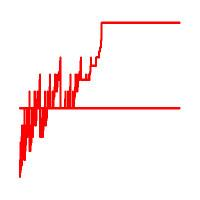A plot of the maximum-digit-average function for binary representations.2003

• Distributional Analyses of Euclidean Algorithms and Euclidean Algorithms are Gaussian with Viviane Baladi. The first (Distributional Analysis) is a front end to the second (...Gaussian), which is the more detailed journal submission, replete with proofs. Take your favorite Euclidean algorithm, one that computes GCD's, like the standard, odd-quotient, or neareast-integer brand. Choose your favorite "cost" measure like the number of iterations, the number of occurrences of your birthdate, the number of times a quotient (digit) that is a prime occurs, and so on. Then, over fractions p/q with denominator less than a fixed bound, the distribution of this cost functional is asymptotically Gaussian. This vastly generalizes an earlier result of Doug Hensley who proved the theorem in the case of the standard algorithm and the number of iterations. Also we obtain optimal error terms. That was tricky, and Viviane and I had to prove the existence of a pole-free strip for certain pseudo-Dirichlet series by suitably extending subtle estimates due to Dolgopyat. Anyhow, I was happy that the dynamical systems and transfer operator approach now gives us access to distributions and to estimates that are as tight as can be for a wide variety of parameters.
Distributional analysis... has been presented at the ANALCO'04 meeting in New Orleans, in January 2004. The document has 15 pages nicely laid out on two columns.
...Gaussian has been submitted to a number theory(?) journal. The version has also been ArXived as cs.DS/0307062 as a 45 page document. (Make sure you get Version #3, revised April 2004.) Makes for perfect bedtime reading!

• Exponential decay of correlations for surface semi-flows without finite Markov partitions, with Viviane Baladi. That's probably the most amazing title of any paper I have ever been author/coauthor of :-). Let me just say here that it presents another approach to estimates of Dolgopyat's type which applies to systems with an infinite number of branches. The need for this arose during our quest for distributional results.
Dated November 2003. To appear in Proceedings of the American Mathematical Society

• Dynamical Analysis of the Parameterized Lehmer-Euclid Algorithm, with Benoit Daireaux. Practitioners of GCD calculations in computer algebra and cryptography are certainly aware of Lehmer's optimization of Euclid's algorithm. Actual computers manipulate words instead of single digits, which is a form of parallelism that can be taken advantage of: simply compute a chunk of the continued fraction expansion of p/q by applying the continued fraction algorithm to the leading words of p and q, proceed with care, and finally adjust. We analyse a version of this idea and obtain very precise estimates, based on--guess what!---dynamical systems.
Some Lectures on Number Theory, Elliptic Curves and Cryptology by Kapil Hari Paranjape: These lecture notes succinctly describe some of the algorithms at stake here.
• Systèmes dynamiques et algorithmique. This started with a mini-course taught at the ALÉA'2002 meeting by Viviane Baladi and myself. Frédéric Chazal, Véronique Maume, and I wrote down lecture notes which have been subsequently carefully scrutinized by Frédéric Chyzak [thanks, Fred!]. Aagh! It's in French. If you read that exotic language, you'll find a summary in 30 pages of the core theory (hopefully) written in an accessible style. You'll strat by an introduction to the dynamics of maps on the interval, density transformers and Markov sources. Branches may or maynot be complete. Each case carries with it an interesting class of transfer operators acting on a suitable functional space. Furthermore, the method of inducing may be used to cope with intermittency phenomena. There's usually a dominant spectral eigenvalue and analytic perturbation theory applies. Bingo! Everything then becomes almost as pleasant as with the usual theory of finite Markov chains. Applications to analysis of algorithms are then sketched. These concern statistical properties of random texts and patterns under quite general source models, dictionaries and digital trees, as well as a wide class of Euclidean and continued fraction algorithms. The text concludes with some open problems (as of 2002) on which progress has been made recently---see also above.
Appears in Algorithms Seminar 2001-2002, F. Chyzak Ed., INRIA Res. Report 5003, November 2003, pp. 121--150.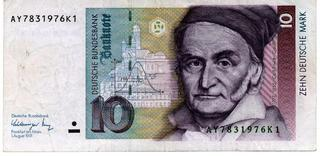The German 10DM banknote with the effigy of Gauss, the discoverer of the (in)famous Bell curve.2002

• Dynamical Analysis of a-Euclidean Algorithms There are perhaps half a dozen ways you may divide 73 by 17: the usual one where quotients are taken by default (i.e., 4), or you may take quotients by excess (5), or to the nearest integer (here 4), or you may impose the remainder to be odd or you may want it to be even. (There are good algorithmic reasons to do so--think of the binary gcd algorithm.) It's surprising that two algorithms that closely resemble one another may exhibit rather different behaviours, e.g., going from linear to quadratic time complexity. We now have a fairly good picture of what goes on, as you may guess by reading these pages. Here, we consider what has been nicknamed the "Japanese" g.c.d. algorithm, which is defined by the constraint that the remainder should always be taken in an interval [a-1,a]. The corresponding problems become trickier as the image below suggests: there the algorithm is seen as moving a window of the class of transformations associated with the usual continued fraction map. This leads to somewhat hairy dynamical systems with incomplete branches, and even a phase transition that it might be interesting to study further...
Joint work with Jérémie Bourdon and Benoit Daireaux. Journal of Algorithms, Vol. 44, No. 1, Jul 2002, pp. 246-285.
• Erratum to: "Dynamical sources in information theory : fundamental intervals and word prefixes" . Errare humanum, perseverare diabolicum. So, the (long) paper cited in the title of this erratum erred, as it omitted a certain technical condition related to distortion in expanding maps of the interval. (Don't panic: the main results of the old paper stand, it's just that their precise domain of validity needs to be amended a bit.) Thanks to good friends in Dijon, Veronique and Frédéric, this could be fixed. I have put this erratum here, not only to get the record straight, but also because aficionados may appreciate the general discussion of suitable functional spaces that accompanies it.
Joint work with Frédéric Chazal and Veronique Maume. To appear in Algorithmica, 2003.
• Hidden Word Statistics. You find your name "hidden" in a medieval treatise on alchemy, say nicely aligned along the diagonal of a page. Does this "mean" something? Well, the answer is (maybe) contained in this paper and its junior companion, Hidden Patterns Statistics, which is a shorter conference version described below. Here, you'll find that the law of a hidden word in a random text is almost certainly Gaussian, with explicit expressions for the mean, the variance, and a few goodies related to the de Bruijn graph, which finds itself highjacked for the purpose of the present research.
Joint work with Philippe Flajolet and Wojtek Szpankowski. Submitted to Journal of the A.C.M. (fall 2002).
• Generalized Pattern-Matching Statistics. Under most current practices, pattern-matching is analysed by enumerative combinatorics of words or by finite-state Markov chains. Here, we depart from this framework and show how, statistically, occurrences of patterns can be predicted with great accuracy in a diversity of models. What I find pleasing about this paper is the simplicity of the conceptual framework, entirely based on density transformers. Also, it applies to a wide range of source models (including memoryless aka Bernoulli and Markov, but also many more) and to a general notion of patterns. There's a catch, though. Only the mean and variance are characterized at this level of generality. I hope to report soon on some of the corresponding distributional analyses (no wonder, the distributions have to be asymptotically normal, but that's not so easy to prove!).
Joint work with Jérémie Bourdon. Appears in Mathematics and Computer Science II: Algorithms, Trees, Combinatorics and Probabilities, Proceedings of Versailles Colloquium, Birhäuser Verlag, 2002, pp. 249-265.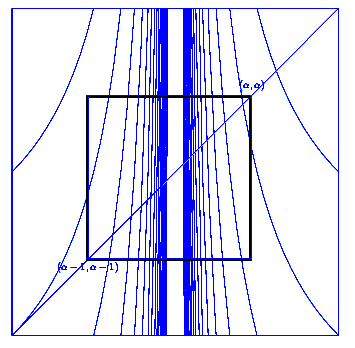Some Euclidean algorithms are fast, others are slow. The question really is Why?.2001

• Hidden Pattern Statistics. A pattern is "hidden" in a text if it appears somewhere in the text, but the letters of the pattern do not necessarily occur contiguously. For instance Brigitte is "hidden" inside the following sentence of Hamlet: "Bring with thee airs from heaven or blasts from hell,..." How significant is this? [guess!] Well, here we characterise the distribution of the number of occurrences of a fixed pattern in a random text, and show that it is asymptoticaly normal. Thus, you can set your own thresholds in order to decide what is meaningful and what is not. The distances between letters may be constrained or not, and still the law has to be gaussian! The problem was initially motivated by intrusion detection in computer security, but is general enough that it should be of [some?] relevance to computational biology. The analysis is, I think, an amusing mix of combinatorial, analytic, and probabilistic methods.
Joint work with Philippe Flajolet, Yves Guivarc'h, and Wojtek Szpankowski. In Proceedings of ICALP'01, Crete, July 2001. Lecture Notes in Computer Science, Vol. 2076, pp. 152--165.Hidden words are everywhere to be found. But what does finding a particular hidden word really mean?2000

• Average-bit Complexity of Euclidean Algorithms. So far, most of my research had been dedicated to somewhat abstract measures of computational complexity, with complexity being measured in the number of arithmetic operations irrespective of the operands' sizes. In this (and the following paper), what is addressed is the Boolean cost, where every bit operation counts. Well, it turns out that techniques based on functional analysis and on classical methods from the analysis of algorithms (e.g., the enumerative uses of regular expressions) combine nicely and open access to the average bit complexity of Euclidean algorithms. It appears for instance that a standard gcd computation performed on binary numbers is hardly more costly than a naive multiplication. True, this is essentially known to practitioners, but it is the first time, I believe, that such complete analyses at bit-complexity level are proposed.
(Joint paper with Ali Akhavi. Appears in the Proceedings of ICALP'2000, Geneva, July 2000. Lecture Notes in Computer Science, volume 1853, pp. 374-87.)
• Digits and Continuants in Euclidean Algorithms. What this paper does is to analyse various characteristics of continued fraction expansion of rational numbers. Analysis of algorithms is still lurking in the background but is not the primary focus. Here, I give new results on the lengths of various encodings of rational numbers by continued fraction representations. Some of these based on Elias or Shannon-Fano codes even appear to be very close to the information-theoretic optimum, with less than a 3% loss.
(Appears in the special issue of Journal de Theorie des Nombres de Bordeaux dedicated to Jacques Martinet on the occasion of his retirement, JTNB 12 (2000) pp 531-570)
• A Unifying Framework for a Class of Euclidean Algorithms (short). and Dynamical Analysis of a Class of Euclidean Algorithms (long). . In the short version, you'll find as promised a unifying framework for the analysis of eight (yes, eight!) Euclidean algorithms--all this condensed in twelve(!) pages. There's even more in the long version... The production chain starts with certain generating functions of costs (of the mixed Dirichlet and ordinary type), relates them to Ruelle operators and their spectral properties, then concludes about averages and moments of costs by means of a suitable Tauberian theorem. The algorithms, some old and some new, appear to split into two categories---the slow ones and the fast ones---and this is eventually related to simple contraction properties of fixed point of certain maps of the interval.
(Paper presented at the conference LATIN'2000. Published by Springer Verlag in Lecture Notes in Computer Science, vol. 1776, Gonnet, Panario and Viola Editors. pp. 343-354. Longer version submitted to Theoretical Computer Science, June 2000.)
• Continued Fractions, Comparison Algorithms, and Fine Structure Constants. How to compare two fractions or equivalently determine the sign of a 2x2 determinant? Although this may sound like a silly question, there are good and bad algorithms when measured either in terms of numerical accuracy or in terms of computational cost. Here is an étude in the mathematics involved in the basic HAKMEM algorithms already discussed in these pages (see year 1998) and in its generalization to sorting. In this largely expository paper, we take the reader through a light tour of dynamical systems (symbolic dynamics), number theory (continued fractions), special functions (multiple zeta values), functional analysis (transfer operators), numerical analysis (series acceleration), and complex analysis (the Riemann hypothesis). These domains all eventually contribute to a detailed characterization of the complexity of comparison and sorting algorithms, either on average or in probability.
(Joint work by Philippe Flajolet and Brigitte Vallée. Appears in Proceedings of the Canadian Mathematical Society, volume titled ``Constructive, Experimental, and Nonlinear Analysis'' in the honour of Jonathan Borwein; Vol 27 (2000) pp 53-82.)
• On the Stack-Size of General Tries. This paper is relative to digital trees (aka tries), as discussed below in relation to earlier papers. Say you're looking for some information in a tree. It is well known that the storage cost of a recursive traversal coincides with the height of the tree. However, the recursive traversal is suboptimal in terms of storage since it keeps track of subtrees to which it will never need to come back. A well-known transformation of the traversal algorithm into an optimized nonrecursive form yields a stack-based algorithm. It is precisely the goal of this paper to analyse the corresponding stack-size on average and in distribution in the case of digital trees. As you might guess, the analysis can be carried out under rather general assumptions on the source that produces digits. In particular, for a binary alphabet and an unbiased source, the reduction in storage consumption is by a factor of log(3)/log(2), that is, about 1.58496.
Joint paper with Jérémie Bourdon and Markus Nebel. In Les cahiers du GREYC,2000, n°4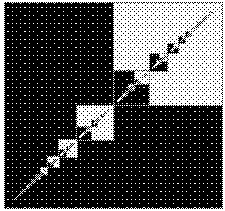Fundamental squares are fundamental when comparing two fractions, but also occasionally in computational geometry!1999

• Dynamical sources in information theory : fundamental intervals and word prefixes. Information theory deals with the way sources produce sequences of symbols (digits, letters) and with the associated metric phenomena. Traditional applications, following Shannon, are in the area of error correction and data compression. This paper shows how a number of characteristics of sources can be analysed precisely in a unified framework based on expanding maps of the interval and their companion transfer operators. A special role is played by the thinning process that successively refines a partition of the interval by appending a new digit. The approach presented has some benefits. It unifies the memoryless models (where letters are drawn independently according to a common probability distribution), the Markovian models (that allow bounded correlations between letters), and a wide class of sources with unboundedly correlated digits, like the digits of continued fraction expansions. This allows for a general treatment of a wide class of sources and it eventually leads, as shown in the next paper listed, to a simple abstract analysis of digital trees.
In Algorithmica 29(1/2), 2001, pp. 262-306.
• Dynamical Sources in Information Theory: A General Analysis of Trie Structures. What is a digital tree, also known in computer science jargon as a "trie"? Well, assume you want to store a dictionary of the Hittite language. You can group together all the words that start with an A and put them in a separate fascicle. No need to repeat the initial A, of course. Then you do the same with B, C, &c. If you proceed recursively (on the second letter, and so on), you transform your set of words composing the dictionary into a tree: this is precisely a digital tree. Such trees have been known for a long time (starting with Fredkin in 1959) to be an efficient data structure. The present paper shows the role of fundamental intervals and word prefixes (as explored in the previous paper) in providing in easy access to probabilistic properties of randomly generated tries. In particular, fundamental constants, like the entropy and the probability of [digit] coincidence, themselves related to spectral properties of transfer operators are main characters of the story. A consequence of the present study is an abstract analysis of tries that encompasses most existing results on height, size, and path length and even gives a bit more!
Joint paper with Julien Clément and Philippe Flajolet; In Algorithmica 29(1/2), 2001, pp. 307-369.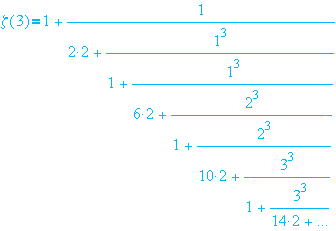Apéry's famous continued fraction for zeta(3). What is a typical contnued fraction like? And what does it have to say about algorithms?1998

• Average-case analyses of three algorithms for computing the Jacobi symbol. The Jacobi symbol is a very important tool in algebra, since it is related to quadratic characteristics in modular arithmetics. Interest in its efficient computation is now reawakened with its utilization in primality tests or more generally in cryptography. All the efficient algorithms that compute the Jacobi symbol have a structure similar to that of the GCD's algorithm, but they involve a modified division-remainder process and are associated with continued fractions of a special type. Three major algorithms (the "Odd", the "Even", and the "Ordinary" algorithm) are considered and are completely analysed in the average case. It appears that their average-case behaviour is very much linked to the analytic properties of the class of linear fractional transformations involved. Two of the algorithms, (the Odd Algorithm and the Ordinary Algorithm) have, as anticipated, an average-case complexity that is O(logN). while the Even Algorithm has average cost O(log(N)^2), which is similar to the GCD subtractive algorithm. This last surprising property is linked to the fact that the corresponding transformation involves a weakly repulsive point. Transfer operators and the method of "inducing" play a role in this analysis, and, naturally, precise constants accompany order-of-growth estimates.
(Joint work with Charly Lemée, October 1998.)

• Dynamique des fractions continues à contraintes périodiques [in French]. The golden section has all its continued fraction quotients equal to 1, while the pattern for e-2 is 1,1,2,1,1,4,1,1,6, etc. What do numbers that satisfy similar periodic constraints look like? Here, I deal with arbitrary sets of periodic constraints. These sets are thus analogous to the classical Cantor sets, where some digit in a base representation is excluded. This paper determines the Hausdorff dimension of constrained reals and the asymptotic density of the corresponding rational or quadratic irrational numbers. Other applications regarding these Cantor-like sets include the analysis of the mean height of continued fraction representations (the cost of Euclid's algorithm) as well as the estimation of the mean value of Lévy's constant for quadratic irrationals.
(In Journal of Number Theory 72(1998), no. 2, 183--235.)

• Continued Fraction Algorithms, Functional Operators, and Structure Constants,by P. Flajolet and B. Vallée. A large amount of research has been devoted to the analysis of metric and probabilistic properties of continued fractions since Gauss first guessed the stationary regime of the continued fraction transformation. This range of problems has been one of the original sources of ergodic theory, with names like Paul Lévy, Kuzmin, or Khinchin. New perspectives then opened when Dieter Mayer, starting in the mid 1970's, discovered that the nascent theory of transfer operators initiated by Ruelle could be successfully applied to this range of problems. In this paper we survey in elementary terms the analysis by transfer operators of a number of basic algorithms, including: the continued fraction and the Euclidean GCD algorithm, the HAKMEM algorithm for comparison of real numbers and 2-dimensional lattice reduction, as well as the digital tree ("trie") built on continued fraction representations. It is amusing to note that the behaviour of the continued fraction tree involves tiny fluctuations whose nature is related to the nontrivial zeros of the classical zeta function and to the Riemann hypothesis.
(The full paper appears in Theoretical Computer Science, March 1998, vol 194 (1-2), pp. 1-34. The version offered here is a preliminary one that was the text of an invited lecture at the 7th conference "Fibonacci Numbers and Applications", Graz, July 1996.)
• Related links. The famous HAKMEM Memorandum, from a team at MIT in 1972; see especially the part that deals with continued fractions. Doug Hensley's home page that contains a version his paper "The Number of Steps in the Euclidean algorithm", where he proves this number to be asymptotically Gaussian. The home page of my coauthor, Philippe Flajolet.

• Dynamics of the Binary Euclidean Algorithm: Functional Analysis and Operators. Computing greatest common divisors (GCD's) lies at the heart of many problems of computational number theory and computer algebra. Perhaps the most efficient algorithm and the one used most often in the case of integers is the binary GCD algorithms. This algorithms is based on subtraction and elimination of powers of two. No multiprecision division is thus needed and the algorithm goes well with binary computers: its cost on binary hardware appears to be barely higher than that of a single division. Richard Brent outlined in 1976 the principles of a heuristic analysis that appeared to match the facts quite well. However, the problem of finding an exact and rigorous average-case analysis has remained hitherto unsolved. In this paper I come up with a complete analysis that is based the introduction of suitable functional spaces and operators. The developments are nontrivial owing to the presence of both singularities and periodicities. I prove in particular that the expected number of steps of the binary GCD algorithm is of the form A.logN where the interesting constant A is discussed in the the latest edition of The Art of Computer Programming, Volume 2, by Don Knuth.
(The final paper appears in Algorithmica, 22 (1998), no. 4, 660--685.)

• The Analysis of Hybrid Trie Structures [HTML], by Julien Clément, Philippe Flajolet, and Brigitte Vallée. In 1997, Bentley and Sedgewick revisited the classical digital tree ("trie") structure that has so many applications in the processing of textual data. One of the problems with the basic form of tries is that, for large alphabets, the branching degree tends to become high, so that search time degrades appreciably while the storage cost due to pointers becomes prohibitive. A simple way to remedy these problems consists in defining a hybrid tree structure where alphabetic searches at each node of the trie are guided by a binary search tree. Bentley and Sedgewick demonstrated the practicality of this approach by means of performance measurements on actual data. The hybrid character of these trees (called "ternary search trees" in the new edition of Sedgewick's Algorithms) poses a challenge to analysts. In this paper, we provide a complete analysis of hybrid tries as well as a theoretical model that makes it possible to compare them with alternative implementations (like list-tries or array-tries). The theoretical predictions match convincingly measurements on textual data (the novel Moby Dick, by Herman Melville!). They justify the fact that hybrid tries lead to an improvement in time complexity by a typical factor of 3. (In Proceedings of the Ninth ACM_SIAM Symposium on Discrete Algorithms (SODA'98), San Francisco, January 1998, pp. 531-539. The form available here is INRIA RR3295, November 1997, 9 pages.)
• Related links. A summary by Julien Clément in the Algorithms Seminar at INRIA, 1997-98. The original papers of Bentley and Sedgewick entitled Ternary Search Trees and Fast Algorithms for Sorting and Searching Strings (Dr Doob's Journal and SODA'97). The home pages of my coauthors, Julien Clément and Philippe Flajolet."Moby Dick" serves to compare theoretical estimates to simulations in the case of trie algorithms.One of Devroye's random tree simulations.1997

• Opérateurs de Ruelle généralisés et analyse en moyenne des algorithmes d'Euclide et de Gauss [in French]. The main theme of this paper is: What happens when the Euclidean algorithm or the Gaussian algorithm for lattice reduction are subjected to nonuniform data? Here, I treat the case of a general real-analytic density. The standard framework of Ruelle operators no longer applies. I then generalize Ruelle operators in such a way that they transform analytic functions of two complex variables. This new generalization gives rise to operators that are better suited to fine-grain analysis of iterates of the continued fraction transformation, while their complete spectrum can be related to that of the classical Ruelle-Mayer operator. As a consequence, very precise characterizations of the complexity of both algorithms under nonuniform input models are derived. (Preliminary version of Acta Informatica, LXXXI.2 (1997), pp. 101-144.)
• Dynamical Systems and the Analysis of General Tries . Digital trees or tries are a fundamental data structure that implements dictionary built on words or the digital structure of data. They support efficiently insertions, deletions, and all sorts of queries, including partial match. (For these reasons they have been used in the computerization of the Oxford English Dictionary.) With the exception of Devroye's work who considered a general density model, most analyses have focussed on fairly simple randomness models, like Bernoulli or Markov. I introduce here a new framework that is capable of dealing with (unboundedly) correlated digits in information sources. Under this framework, the main characteristics of digital trees, namely, height, size, or path length, are precisely analysed.
(This paper was presented at the RALCOM'97 meeting in Santorini Island (!), Greece, October 1997.)
• An average-case analysis of the Gaussian algorithm for lattice reduction, by H. Daudé, P. Flajolet, and B. Vallée. A two-dimensional lattice has infinitely many bases. However only one of these bases has a "nice" aspect and is formed with nearly orthogonal and reasonably small vectors --the reduced basis. Lattice reduction aims to find such a basis. The problem intervenes in many questions of number theory (e.g., the reduction of quadratic forms) and Gauss proposed an essentially optimal algorithm based on a modified continued fraction algorithm. The worst case of this reduction algorithm is logarithmic in the size of the input vectors. Here , we prove that the average case number of steps is asymptotically constant and that the probability of executing a large number of reduction steps decays exponentially. Our treatment characterizes the behaviour of the algorithm in terms of a conditional invariant measure that is related to the transfer operator of classical continued fractions. In a way, analytically, the Gaussian algorithm is like a version of the GCD algorithms that would "leak" (!).
(Appears in Combinatorics, Probability and Computing 6 (4), 1997, pp. 397-433; the version available here is a preliminary one: INRIA, RR 2798, February 1996.)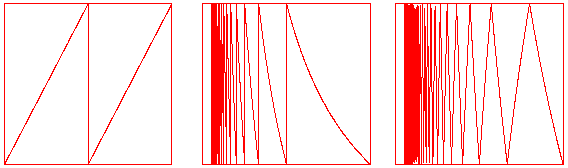Three transformations of the interval iterate to give rise to binary representations, standard continued fractions, and centred continued fractions.Back to my Home Page.